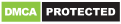### Write Comment

Type in
(Press Ctrl+g to toggle between English and the chosen language)

•Statements : T $geq$ V ; V > M ; M = F Combining above statements, we get : $Tgeq V>M=F$ Conclusions :  I. T # M : $T>M$ = true II. T @ F : $Tgeq F$ = false Thus, only conclusion I is true. => Ans - (A)Powered By:Omega Web Solutions